# AP SSC 10th Class Maths Solutions Chapter 8 Similar Triangles Ex 8.1

AP State Board Syllabus AP SSC 10th Class Maths Textbook Solutions Chapter 8 Similar Triangles Ex 8.1 Textbook Questions and Answers.

## AP State Syllabus SSC 10th Class Maths Solutions 8th Lesson Similar Triangles Exercise 8.1

### 10th Class Maths 8th Lesson Similar Triangles Ex 8.1 Textbook Questions and Answers

Question 1.
In △PQR, ST is a line such that $$\frac{PS}{SQ}$$ = $$\frac{PT}{TR}$$ and also ∠PST = ∠PRQ.
Prove that △PQR is an isosceles triangle.
Given : In △PQR,
$$\frac{PS}{SQ}$$ = $$\frac{PT}{TR}$$ and ∠PST= ∠PRQ.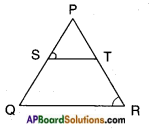R.T.P: △PQR is isosceles.
Proof: $$\frac{PS}{SQ}$$ = $$\frac{PT}{TR}$$
Hence, ST || QR (Converse of Basic proportionality theorem)
∠PST = ∠PQR …….. (1)
(Corresponding angles for the lines ST || QR)
Also, ∠PST = ∠PRQ ……… (2) given
From (1) and (2),
∠PQR = ∠PRQ
i.e., PR = PQ
[∵ In a triangle sides opposite to equal angles are equal]
Hence, APQR is an isosceles triangle.Question 2.
In the given figure, LM || CM and LN || CD. Prove that $$\frac{AM}{AB}$$ = $$\frac{AN}{AD}$$.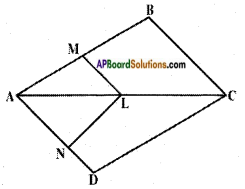Given : LM || CB and LN || CD In △ABC, LM || BC (given) Hence,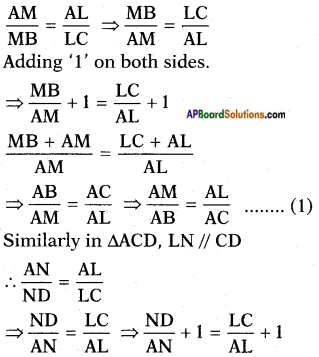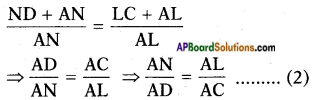From (1) and (2)
∴ $$\frac{AM}{AB}$$ = $$\frac{AN}{AD}$$.

Question 3.
In the given figure, DE || AC and DF || AE. Prove that $$\frac{BF}{FE}$$ = $$\frac{BE}{AC}$$.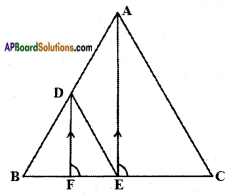In △ABC, DE || AC
Hence $$\frac{BE}{EC}$$ = $$\frac{BD}{DA}$$ ………. (1)
[∵ A line drawn parallel to one side of a triangle divides the other two sides in the same ratio – Basic proportionality theorem]
Also in △ABE, DF || AE
Hence $$\frac{BF}{FE}$$ = $$\frac{BD}{DA}$$ ………. (2)
From (1) and (2), $$\frac{BF}{FE}$$ = $$\frac{BE}{AC}$$ Hence proved.Question 4.
Prove that a line drawn through the mid-point of one side of a triangle parallel to another side bisects the third side (Using Basic proportionality theorem).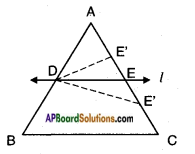Given: In △ABC; D is the mid-point of AB.
A line ‘l’ through D, parallel to BC, meeting AC at E.
R.T.P: E is the midpoint of AC.
Proof:
DE || BC (Given)
then
$$\frac{AD}{DB}$$ = $$\frac{AE}{EC}$$(From Basic Proportional theorem)
Also given ‘D’ is mid point of AB.
⇒ $$\frac{AD}{DB}$$ = $$\frac{DB}{DB}$$ = $$\frac{AE}{EC}$$ = 1
⇒ AE = EC
∴ ‘E’ is mid point of AC
∴ The line bisects the third side $$\overline{\mathrm{AC}}$$.
Hence proved.

Question 5.
Prove that a line joining the mid points of any two sides of a triangle is parallel to the third side. (Using converse of Basic proportionality theorem)
Given: △ABC, D is the midpoint of AB and E is the midpoint of AC.
R.T.P : DE || BC.
Proof: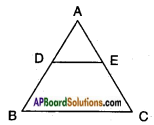Since D is the midpoint of AB, we have AD = DB ⇒ $$\frac{AD}{DB}$$ = 1 ……. (1)
also ‘E’ is the midpoint of AC, we have AE = EC ⇒ $$\frac{AE}{EC}$$ = 1 ……. (2)
From (1) and (2)
$$\frac{AD}{DB}$$ = $$\frac{AE}{EC}$$
If a line divides any two sides of a triangle in the same ratio then it is parallel to the third side.
∴ DE || BC by Basic proportionality theorem.
Hence proved.Question 6.
In the given figure, DE || OQ and DF || OR. Show that EF || QR.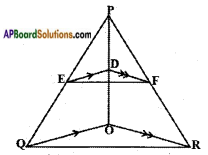Given: △PQR, DE || OQ; DF || OR
R.T.P: EF || QR
Proof:
In △POQ;
$$\frac{PE}{EQ}$$ = $$\frac{PD}{DO}$$ ……. (1)
[∵ ED || QO, Basic proportionality theorem]
In △POR; $$\frac{PF}{FR}$$ = $$\frac{PD}{DO}$$ ……. (2) [∵ DF || OR, Basic Proportionality Theorem]
From (1) and (2),
$$\frac{PE}{EQ}$$ = $$\frac{PF}{FR}$$
Thus the line $$\overline{\mathrm{EF}}$$ divides the two sides PQ and PR of △PQR in the same ratio.
Hence, EF || QR. [∵ Converse of Basic proportionality theorem]

Question 7.
In the given figure A, B and C are points on OP, OQ and OR respec¬tively such that AB || PQ and AC || PR. Show that BC || QR.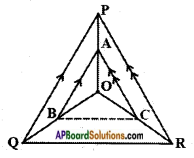Given:
In △PQR, AB || PQ; AC || PR
R.T.P : BC || QR
Proof: In △POQ; AB || PQ
$$\frac{OA}{AP}$$ = $$\frac{OB}{BQ}$$ ……… (1)
(∵ Basic Proportional theorem)
and in △OPR, Proof: In △POQ; AB || PQ
$$\frac{OA}{AP}$$ = $$\frac{OC}{CR}$$ ……… (2)
From (1) and (2), we can write
$$\frac{OB}{BQ}$$ = $$\frac{OC}{CR}$$
Then consider above condition in △OQR then from (3) it is clear.
∴ BC || QR [∵ from converse of Basic Proportionality Theorem]
Hence proved.Question 8.
ABCD is a trapezium in which AB || DC and its diagonals intersect each other at point ‘O’. Show that$$\frac{AO}{BO}$$ = $$\frac{CO}{DO}$$.
Given: In trapezium □ ABCD, AB || CD. Diagonals AC, BD intersect at O.
R.T.P: $$\frac{AO}{BO}$$ = $$\frac{CO}{DO}$$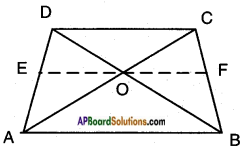Construction:
Draw a line EF passing through the point ‘O’ and parallel to CD and AB.
Proof: In △ACD, EO || CD
∴ $$\frac{AO}{CO}$$ = $$\frac{AE}{DE}$$ …….. (1)
[∵ line drawn parallel to one side of a triangle divides other two sides in the same ratio by Basic proportionality theorem]
In △ABD, EO || AB
Hence, $$\frac{DE}{AE}$$ = $$\frac{DO}{BO}$$
[∵ Basic proportionality theorem]
$$\frac{BO}{DO}$$ = $$\frac{AE}{ED}$$ …….. (2) [∵ Invertendo]
From (1) and (2),
$$\frac{AO}{CO}$$ = $$\frac{BO}{DO}$$
⇒ $$\frac{AO}{BO}$$ = $$\frac{CO}{DO}$$ [∵ Alternendo]Question 9.
Draw a line segment of length 7.2 cm and divide it in the ratio 5 : 3. Measure the two parts.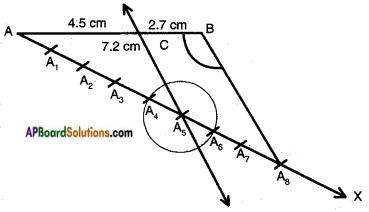1. Draw a line segment $$\overline{\mathrm{AB}}$$ of length 7.2 cm.
3. Mark off 5 + 3 = 8 equal parts (A1, A2, …., A8) on $$\stackrel{\leftrightarrow}{\mathrm{AX}}$$ with same radius.
5. Draw a line parallel to $$\stackrel{\leftrightarrow}{\mathrm{A}_{8} \mathrm{~B}}$$ at A5 meeting AB at C.